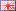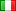Language# Convert : square millimeter to square yard

## How to convert a area : square millimeter to square yard ?

Just enter a value then convert it. Select the decimals number (if you need more than 3 digits after the decimal marker)/area/square_millimeter-to-square_yard